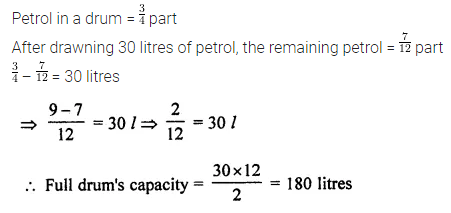# ML Aggarwal Class 7 Solutions for ICSE Maths Chapter 2 Fractions and Decimals Objective Type Questions

## ML Aggarwal Class 7 Solutions for ICSE Maths Chapter 2 Fractions and Decimals Objective Type Questions

Mental Maths

Question 1.
Fill in the blanks:
(i) In fractions with same numerator, the fraction with greater denominator is …….
(ii) $$\frac { 114 }{ 138 }$$ reduced to simplest forms is ………
(iii) $$\frac { 154 }{ 286 }$$ = $$\frac { …… }{ 13 }$$
(iv) The reciprocal of the fraction 2$$\frac { 3 }{ 8 }$$ is …….
(v) There are …….. minutes in $$\frac { 2 }{ 5 }$$ th of 2 hours.
(vi) 2$$\frac { 3 }{ 7 }$$ × 4$$\frac { 2 }{ 3 }$$ = …….
(vii) 1$$\frac { 2 }{ 3 }$$ ÷ 2$$\frac { 1 }{ 5 }$$ = ………
(viii) If the price of 7 similar pens is ₹ 37.80, then the price of each pen is ……..
(ix) 5.4 × 2.35 = ……
(x) 0.32 ÷ 8 = …….
(xi) 45 mg = ………. g
(xii) 5.06 kg = ……… kg …….. g
(xiii) 7.035 m = ……. m …….. cm …….. mm
(xiv) The product of a proper fraction and an improper fraction is …… the improper fraction.
(xv) The lowest form of the product 2$$\frac { 3 }{ 7 }$$ × $$\frac { 7 }{ 9 }$$ is ………
(xvi) Ravi ate $$\frac { 2 }{ 7 }$$ part of a cake while his sister Rani ate $$\frac { 4 }{ 5 }$$ of the remaining. Part of the cake left is ……
Solution: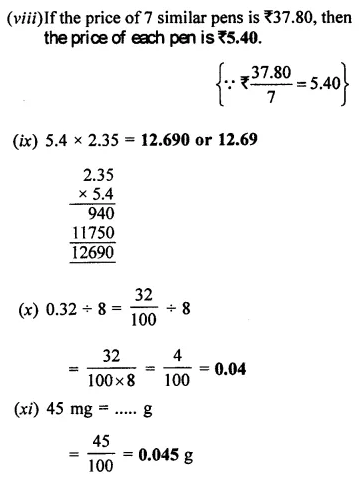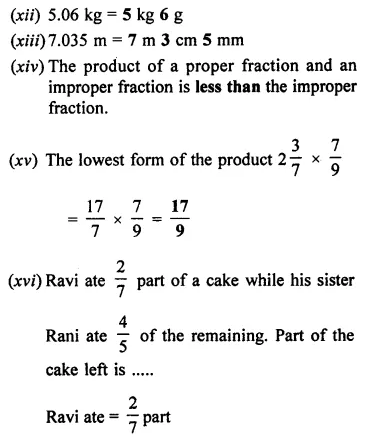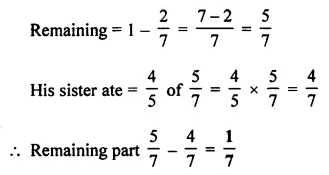Question 2.
State whether the following statements are true (T) or false (T):
(i) The reciprocal of 1 is 0.
(ii) The reciprocal of a proper fraction is a proper fraction.
(iii) The reciprocal of an improper fraction is an improper fraction.
(iv) Product of two fractions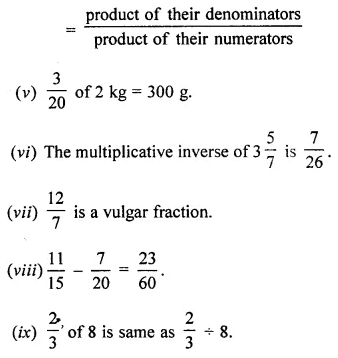(x) The product of two proper fractions is greater than each of the two fractions.
(xi) To multiply a decimal number by 10, move the decimal point to the left by one place.
(xii) To divide a decimal number by 100, move the decimal point to the left by two places.
Solution: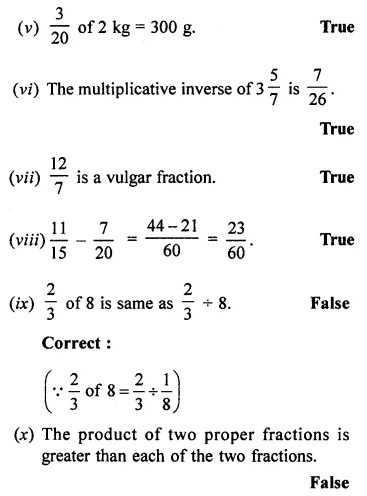Multiple Choice Questions

Choose the correct answer from the given four options (3 to 20):
Question 3.
$$\frac { 5 }{ 6 }$$ of 480 is
(a) 400
(b) 576
(c) 480
(d) none of these
Solution:Question 4.
The reciprocal of 5$$\frac { 2 }{ 3 }$$ is
(a) 5$$\frac { 3 }{ 2 }$$
(b) 3$$\frac { 2 }{ 5 }$$
(c) 2$$\frac { 3 }{ 5 }$$
(d) $$\frac { 3 }{ 17 }$$
Solution: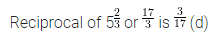Question 5.
The fraction $$\frac { 11 }{ 7 }$$ lies between
(a) 11 and 7
(b) 1 and 2
(c) 0 and 1
(d) 2 and 3
Solution: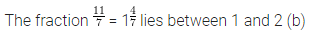Question 6.
If the cost of 1 kg almonds is ₹ 460, then the cost of $$\frac { 2 }{ 5 }$$ kg of almonds is
(a) ₹ 92
(b) ₹ 184
(b) ₹ 230
(d) ₹ 1200
Solution:Question 7.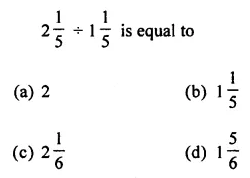Solution: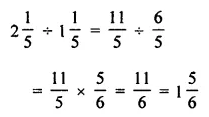Question 8.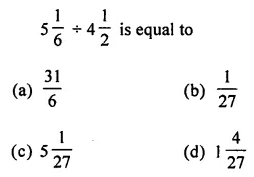Solution: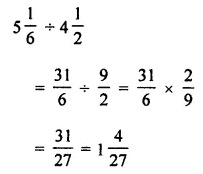Question 9.
If $$\frac { 3 }{ 4 }$$ of a number is 12, then the number is
(a) 9
(b) 16
(c) 18
(d) 32
Solution: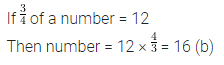Question 10.
Arif bought 3 dozen eggs. He found $$\frac { 1 }{ 9 }$$ of them were rotten. The number of rotten eggs was
(a) 4
(b) 3
(c) 6
(d) 8
Solution: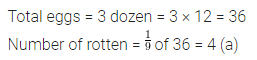Question 11.
Shruti reads a novel for 1$$\frac { 3 }{ 4 }$$ hours daily, if she reads the entire novel in 6 days, then the time she takes to read the entire novel is
(a) 7$$\frac { 1 }{ 2 }$$ hours
(b) 9$$\frac { 1 }{ 2 }$$ hours
(c) 10$$\frac { 1 }{ 2 }$$ hours
(d) 11$$\frac { 1 }{ 2 }$$ hours
Solution:Question 12.
The place value of the digit 7 in the decimal number 35.0471 is
(a) 7
(b) $$\frac { 7 }{ 100 }$$
(c) $$\frac { 1 }{ 1000 }$$
(d) $$\frac { 7 }{ 1000 }$$
Solution:Question 13.
0.002 × 0.3 is
(a) 0.6
(b) 0.06
(c) 0.006
(d) 0.006
Solution:Question 14.
The value of the mixed fraction 5$$\frac { 3 }{ 8 }$$ is
(a) 5.735
(b) 5.375
(c) 5.625
(d) 5.875
Solution:Question 15.
0.35 ÷ 0.7 is
(a) 50
(b) 5
(c) 0.5
(d) 0.05
Solution:Question 16.
30 m 5 cm is same as
(a) 30.5 m
(b) 3.05 m
(c) 30.05 m
(d) 30.005 m
Solution:Question 17.
0.05309 × 1000 is
(a) 5.309
(b) 53.09
(c) 530.9
(d) none of these
Solution: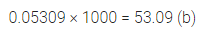Question 18.
2.305 ÷ 1000 is
(a) 0.2305
(b) 0.02305
(c) 0.002305
(d) none of these
Solution:Question 19.
If each side of a regular hexagon is 3.5 cm, then the perimeter of the hexagon is
(a) 17.5 cm
(b) 21 cm
(c) 18.5 cm
(d) 24.5 cm
Solution:Question 20.
Which of the following numbers has the smallest value?
(a) 0.0002
(b) $$\frac { 2 }{ 1000 }$$
(c) 0.02 × 0.001
(d) $$\frac { 2 }{ 1000 }$$ ÷ 0.01
Solution:Value Based Questions

Question 1.
Seema had one sandwich for her lunch and her two other friends in the class forgot to bring their lunch. Seema decided to share equally her sandwich with her friends. How much part of the sandwich each girl will get? What have you learnt by this behaviour of Seema?
Solution:Question 2.
A farmer had a large field of dimensions 1396.5 m by 272.72 m. Find the area of the field. He left $$\frac { 1 }{ 6 }$$ of this area to make a playground for the children of the locality. Find the area of the playground. Which value of the farmer is reflected in this act?
Solution: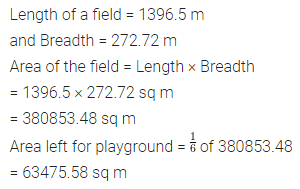Higher Order Thinking Skills (HOTS)

Question 1.
I read $$\frac { 4 }{ 9 }$$ of a book on one day and $$\frac { 3 }{ 5 }$$ of the remaining next day. If 100 pages of the book were still left unread, how many pages did the book contain?
Solution: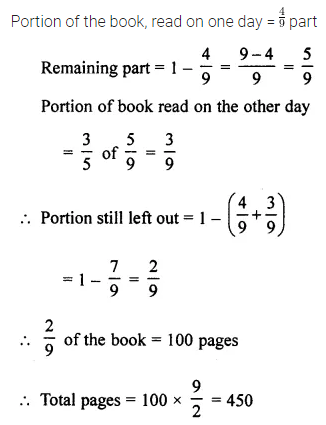Question 2.
A drum of petrol is $$\frac { 3 }{ 4 }$$ full. When 30 litres of oil are drawn from it, it is $$\frac { 7 }{ 12 }$$ full. Find the capacity of the drum.
Solution: